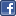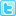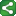Microsoft Excel Resource Center Free Macros, Formulas, Functions, Tutorials, Downloads, Add-Ins & More!
 Monte Carlo Tutorial power4XL Home
 Contents

A Brief Introduction to Monte Carlo MethodsShareThis

Monte Carlo refers to a widely used approach for solving complex problems using computer algorithms to simulate the variables in the problem.  Typically an algorithm is developed to "model" the problem, and then the algorithm is run many times (from a few hundred up to millions) in order to develop a statistical data set for how the model behaves.

For the simplest example, consider the basic coin toss.  This is a process which has two possible outcomes (heads or tails), each with a 50% probability.  In a million coin tosses, roughly half will be "heads" and half will be "tails".  No complex math is required to know this.

A simple Monte Carlo simulation would prove the same result.  If you were to develop a spreadsheet with a random number generator resulting in 0 for "heads" and 1 for "tails" (which we will do later in the tutorial), then cause the spreadsheet to recalculate a million times, each time recording the results in a database, you could then run a report on the database which would reveal that very close to 50% of the recalculations resulted in 0 or "heads" and the other 50% in 1 or "tails".

Taking this a step further, suppose you wanted to know the likelihood of getting "heads" 7 times out of 10 coin tosses.  Again here, statistical mathematical equations provide an accurate answer without the need for Monte Carlo.  But a Monte Carlo spreadsheet could simulate a series of ten coin tosses as easy as a single coin toss, and could keep a tally of how many times 7 "heads" were returned from 10 tosses after running a few thousand iterations of the model.  Analyzing the resulting data should give an answer very close to the mathematical statistical probability of getting 7 "heads".

Now go one step further.  Suppose your commute to work consists of the following:

• Drive 2 miles on a highway, with 90% probability you will be able to average 65 MPH the whole way, but with a 10% probability that a traffic jam will result in average speed of 20 MPH.

• Come to an intersection with a traffic light that is red for 90 seconds, then green for 30 seconds.

• Travel 2 more miles on a surface street, averaging 30 MPH with a standard deviation of 10 MPH.

You want to know how much time to allow for the commute in order to have a 75% probability of arriving at work on time.  And, when you have an important meeting, you want to know how early you need to leave the house in order to have a 99.5% probability of arriving on time.

Though you could conceivably solve this problem using mathematical equations, a problem with this degree of complexity becomes more efficient to solve using a Monte Carlo simulation.

The basic approach would be to build a spreadsheet with random number generators which replicate the probabilities in the problem, and which calculate the total commute time for a particular drive to work.  Each time you recalculate the spreadsheet, the drive times and traffic light delays are generated afresh according to the actual probabilities you have programmed.  By simulating 10,000 trips to the office in a few seconds on your spreadsheet, you can then easily see what the 75th percentile and 99.5th percentile are for your drive time.

That's essentially all there is to it.  The more complex the problem, the better it lends itself to Monte Carlo simulations over mathematical solving.  Many times the problem may involve some guessing at how a particular variable behaves (is it a normal distribution curve, or a linear?), but by using Excel spreadsheets to build your models, it's easy to change the assumptions behind each variable to study the sensitivity the results on each of your assumptions.ShareThis
Prev | Contents | Next

Copyright notice:  This site and all content, including computer code and spreadsheet examples, are copyright 2006 by Fritz Dooley.  License is granted for individual users to download examples and to copy code directly into user's spreadsheets and Visual Basic for Applications files.  Users may not redistribute code in any way.  Providing hyperlinks to this web site is encouraged, but posting code and examples on other web sites is expressly forbidden.  "Microsoft" is a registered trademark of Microsoft Corporation.   Neither this web site nor Fritz Dooley is affiliated with Microsoft Corporation.